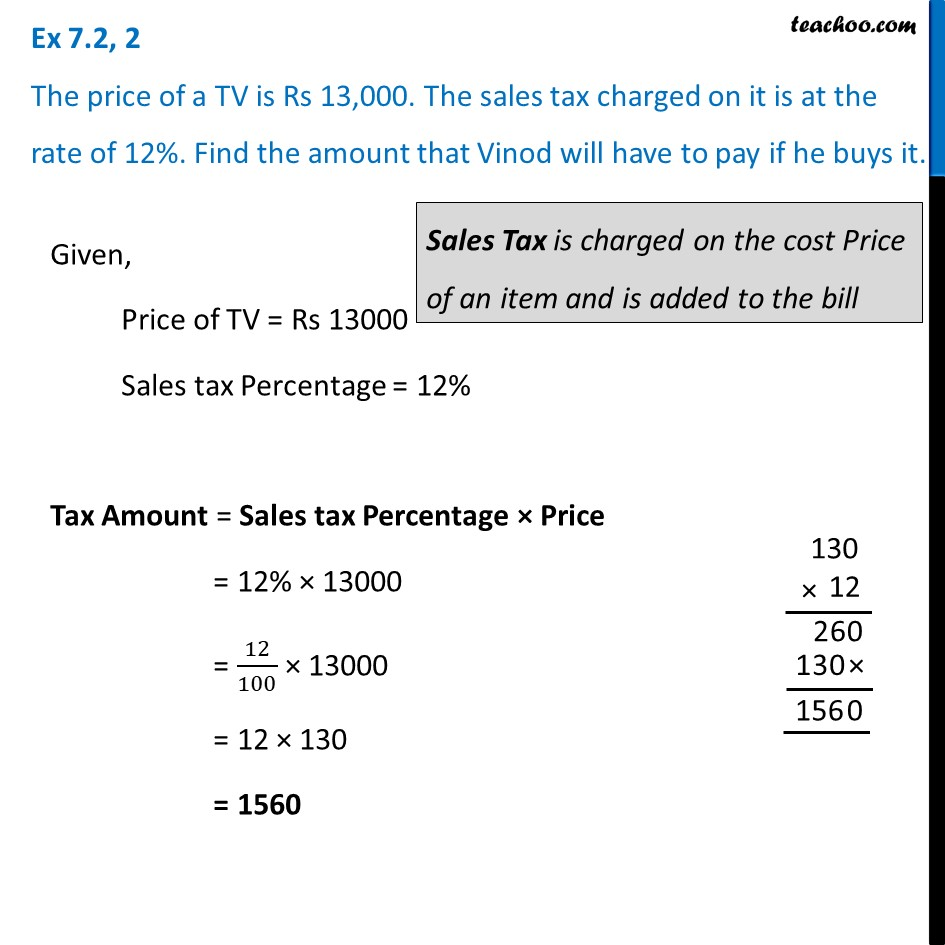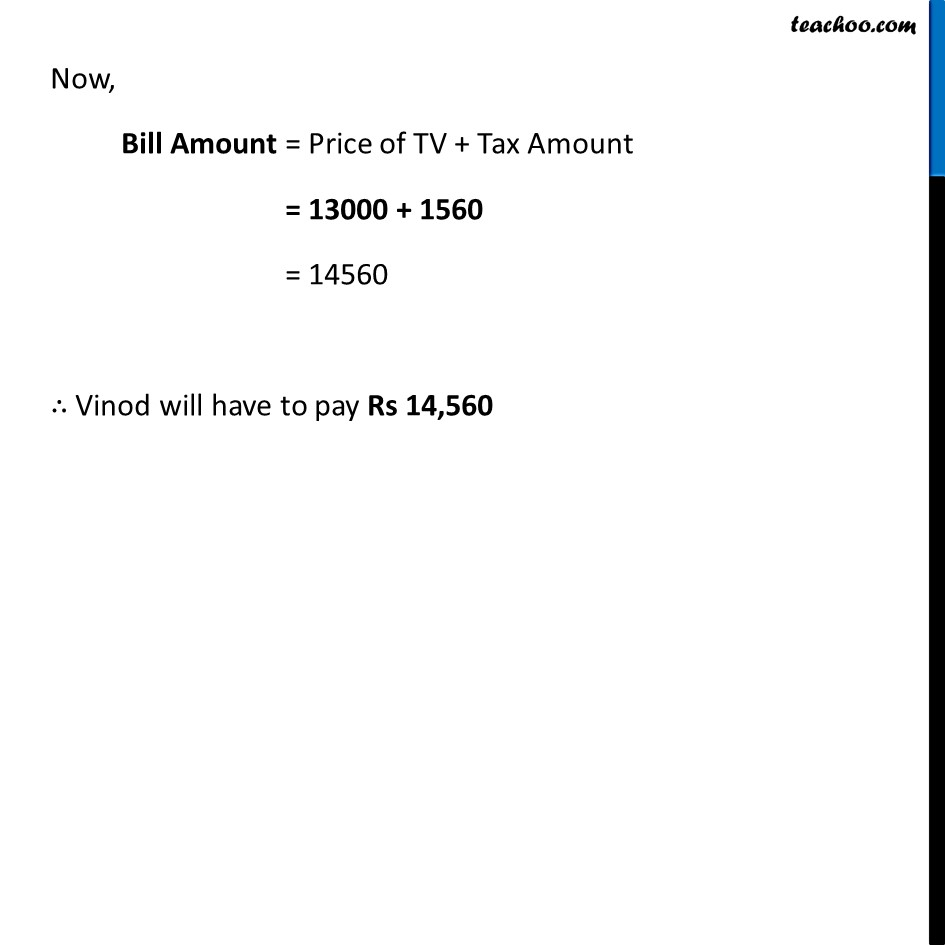Ex 7.2

Chapter 7 Class 8 Comparing Quantities
Serial order wiseLearn in your speed, with individual attention - Teachoo Maths 1-on-1 Class

### Transcript

Ex 7.2, 2 The price of a TV is Rs 13,000. The sales tax charged on it is at the rate of 12%. Find the amount that Vinod will have to pay if he buys it.Given, Price of TV = Rs 13000 Sales tax Percentage = 12% Tax Amount = Sales tax Percentage × Price = 12% × 13000 = 12/100 × 13000 = 12 × 130 = 1560 Now, Bill Amount = Price of TV + Tax Amount = 13000 + 1560 = 14560 ∴ Vinod will have to pay Rs 14,560